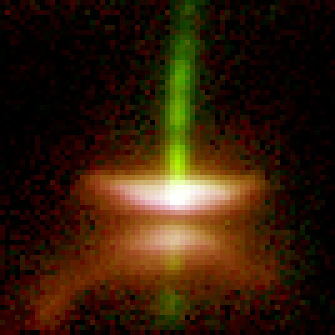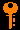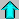# Lecture 12 - Stellar Structure II: Energy Transport (2/23/99)Stellar Structure I --- | --- ISMReading:
Chapter 16-1, 16-2 (ZG4)

Notes:
pages 46 - 51

The Herbig-Haro Object HH32, as seen by HST WFPC2. This is a young star that still has its accretion disk and outflow jet! (Courtesy Chris Burrows, WFPC2 Science Team, NASA)
Key Question:## Investigations:

1. Equations of State
• What is an equation of state?
• What is the equation of state of an ideal gas?
• What the relation between dP/dr and dP/drho?
• Why do we parameterize P=P0*rho^gamma?
2. Energy Generation and Radiative Transport
• What is the luminosity dL generated in a shell of mass dM?
• What is dL/dr in a spherical star?
• For radiative transport of energy, what is dP_rad/dr in terms of dT/dr?
• What is dP_rad/dr in terms of L(r)?
• How does this depend on the opacity, density, T and L(r) at the shell?
3. Convective Energy Transport
• What is the expected scale of convection (ie. the pressure scale height in the interior) in the Sun?
• How does convection transport energy?
• For an ideal gas P=nkT, what is dP/dr in terms of dT/dr and dn/dr (for number density n)?
• What is a polytropic equation of state?
• What is the convective temperature gradient dT/dr for a pressure gradient dP/dr?
• When is a gas stable against convection?
• Where might you expect energy to be carried by convection in a star?
4. Main Sequence Scaling Relations
• How does the mean density scale with mass and radius?
• How does the central pressure scale with mass and radius?
• How does the central temperature scale with mass and radius?
• How does the luminosity scale with mass and opacity?
• Why does this relation depend on the opacity but not the energy generation?
• What is the Kramer's Opacity Law for bound-free and free-free opacity?
• What is electron scattering cross section and opacity?

## More Stellar Structure in Outline:

1. Stellar Structure continued...
• The mass-luminosity relation (L/Lsun) = (M/Msun)^3.5 is an approximation to the solution of the equations of stellar structure for a star of mass close to that of the Sun. The actual exponent ranges from 2.5 to over 4 over the main sequence, and of course does not obey anything like this off the main sequence.
• It turns out that the stellar structure that you find depends only upon the mass M of the star! If the energy is produced by fusion as given by E(r)=E(T(r),rho(r)), then the star falls on the main sequence and the surface temperature T, and luminosity L depend on the mass M such that they increase as you increase the mass.
• Because higher mass stars are much more luminous, they burn their fuel faster, and have shorter lifetimes on the main sequence. Note that the lifetime t is proportional to M/L (fuel/rate of burning) and for stars of mass near the Sun's:

t/tsun = (M/Msun)/(L/Lsun) = M/M^3.5 = 1/M^2.5

Thus, a star of twice the mass of the Sun would live only 18% of the life span of the Sun. A star of 0.5 Msun would live 5.6 times longer.
• The most massive stars live only a few millions of years! (Short by cosmic standards.)Prev Lecture ---Next Lecture ---Astr12 Index ---Astr12 Home

smyers@nrao.edu Steven T. Myers